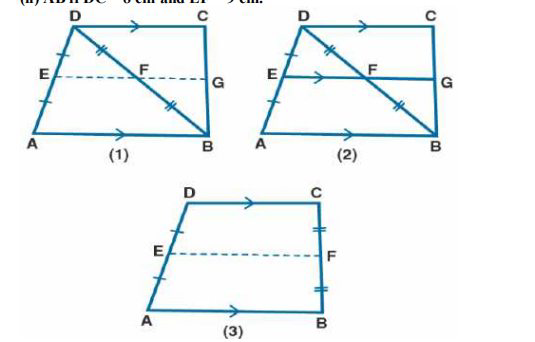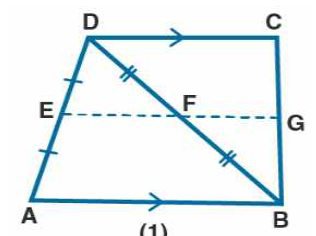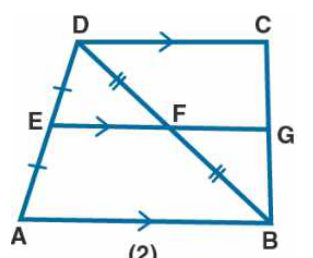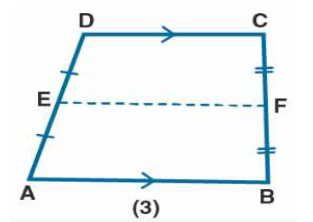# ML Aggarwal Solutions Class 9 Mathematics Solutions for Mid Point Theorem Exercise 11 in Chapter 11 - Mid Point Theorem

(a) In the quadrilateral (1) given below, AB || DC, E and F are the mid-points of AD and BD

respectively. Prove that:

(i) G is the mid-point of BC

(ii) EG = ½ (AB + DC)

(b) In the quadrilateral (2) given below, AB || DC || EG. If E is mid-point of AD prove that:

(i) G is the mid-point of BC

(ii) 2EG = AB + CD

(c) In the quadrilateral (3) given below, AB || DC.

E and F are mid-point of non-parallel sides AD and BC respectively. Calculate:

(i) EF if AB = 6 cm and DC = 4 cm.

(ii) AB if DC = 8 cm and EF = 9 cm.(a) It is given that

AB || DC, E and F are mid-points of AD and BTo prove:

(i) G is mid-point of BC

(ii) EG = ½ (AB + DC)

Proof:

In Δ ABD

F is the mid-point of BD

DF = BF

E is the mid-point of AD

EF || AB and EF = ½ AB ….. (1)

It is given that AB || CD

EG || CD

F is the mid-point of BD

FG || DC

G is the mid-point of BC

FG = ½ DC …….. (2)

EF + FG = ½ AB + ½ DC

Taking ½ as common

EG = ½ (AB + DC)

Therefore, it is proved.

(b) It is given that

Quadrilateral ABCD in which AB || DC || EG

E is the mid-point of ADTo prove:

(i) G is the mid-point of BC

(ii) 2EG = AB + CD

Proof:

AB || DC

EG || AB

So we get

EG || DC

In Δ DAB,

E is the mid-point of BD and EF = ½ AB ….. (1)

In Δ BCD,

F is the mid-point of BD and FG || DC

FG = ½ CD …… (2)

EF + FG = ½ AB + ½ CD

Taking out the common terms

EG = ½ (AB + CD)

Hence, it is proved.

(c) It is given that

A quadrilateral in which AB || DC

E and F are the mid-points of non-parallel sides AD and BCTo prove:

(i) EF if AB = 6 cm and DC = 4 cm.

(ii) AB if DC = 8 cm and EF = 9 cm.

Proof:

We know that

The length of line segment joining the mid-points of two non-parallel sides is half the sum of the lengths of the

parallel sides

E and F are the mid-points of AD and BC

EF = ½ (AB + CD) …… (1)

(i) AB = 6 cm and DC = 4 cm

Substituting in equation (1)

EF = ½ (6 + 4)

By further calculation

EF = ½ × 10 = 5 cm

(ii) DC = 8 cm and EF = 9 cm

Substituting in equation (1)

EF = ½ (AB + DC)

By further calculation

9 = ½ (AB + 8)

18 = AB + 8

So we get

18 – 8 = AB

AB = 10 cm

Related Questions
Exercises

Lido

Courses

Teachers

Book a Demo with us

Syllabus

Maths
CBSE
Maths
ICSE
Science
CBSE

Science
ICSE
English
CBSE
English
ICSE
Coding

Terms & Policies

Selina Question Bank

Maths
Physics
Biology

Allied Question Bank

Chemistry
Connect with us on social media!